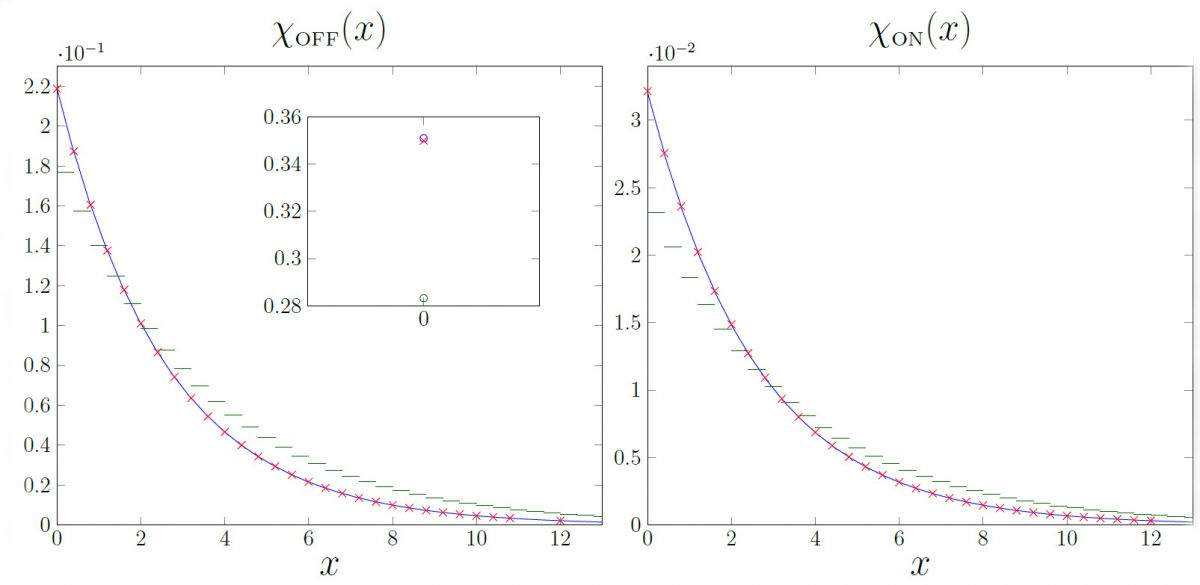# giang-nguyen-figures.jpgComparisons between exact densities (red crosses) and their approximations (blue and green curves) for various scenarios of a stochastic fluid-fluid process. The piecewise-linear approximation (blue curve) fit through all exact values, indicating that the approximation is highly accurate. The subplot inside the left plot shows the corresponding probability masses at value 0, where once again the piecewise-linear approximation (blue circle) takes the same value as the exact probability mass (red cross).